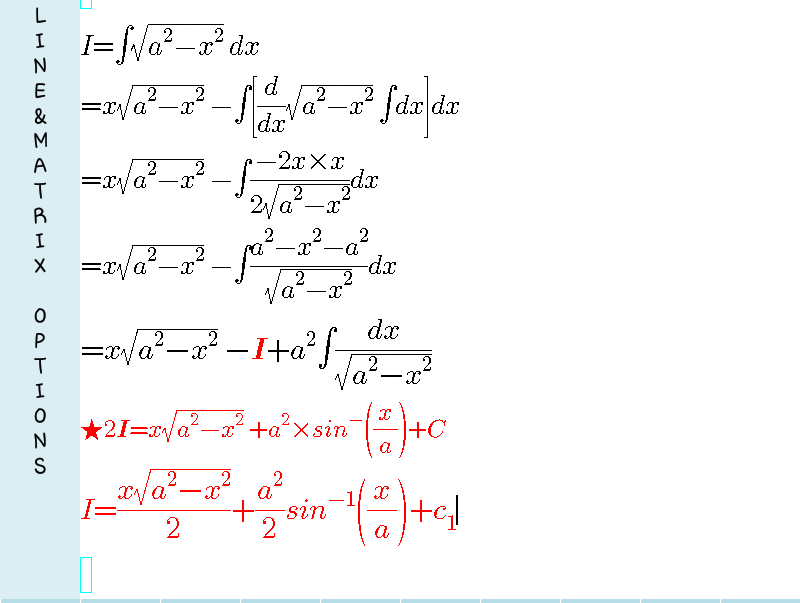# Doubt from integration by parts

√(a^2-x^2)

i solved using trigo substitution and got it , but could not solve by byparts

Take 1st function as √a^2-x^2 and 2nd function as 1.
Proceed this way..yu will get it easily..
Do ask if yu need complete solution

An important hint so that yu can do other steps by yourself ;
After applying IBP ,you will get a term -> integral of -x^2/√a^2-x^2
Convert the Nr of this term to (a^2-x^2-a^2)2 Likes

thank you so much